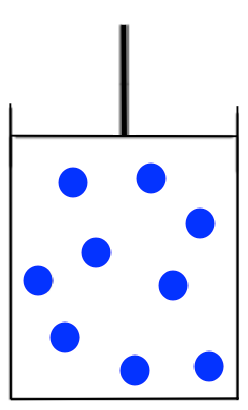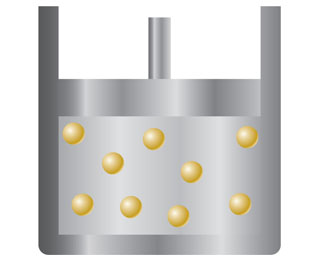# Problem: You have a sample of gas in a container with a movable piston, such as the one in the drawing.Redraw the container to show what it might look like if the temperature of the gas decreases from 300 to 200 K while the pressure is kept constant (assume the gas does not liquefy).

###### FREE Expert Solution

We are asked to redraw the given container to show what it might look like if the temperature of the gas decreases.Recall that Charles Law states that volume and temperature are directly proportional if pressure and moles are constant (i.e. As the volume goes up, the temperature also goes up, and vice-versa):###### Problem Details

You have a sample of gas in a container with a movable piston, such as the one in the drawing.Redraw the container to show what it might look like if the temperature of the gas decreases from 300 to 200 K while the pressure is kept constant (assume the gas does not liquefy).